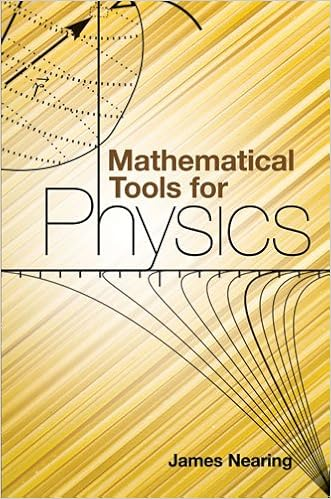## Tuesday, October 13, 2015

### Mathematical Tools for Physics by James NearingHaving the right answer doesn't guarantee understanding. This book helps physics students learn to take an informed and intuitive approach to solving problems. It assists undergraduates in developing their skills and provides them with grounding in important mathematical methods.
Starting with a review of basic mathematics, the author presents a thorough analysis of infinite series, complex algebra, differential equations, and Fourier series. Succeeding chapters explore vector spaces, operators and matrices, multivariable and vector calculus, partial differential equations, numerical and complex analysis, and tensors. Additional topics include complex variables, Fourier analysis, the calculus of variations, and densities and distributions. An excellent math reference guide, this volume is also a helpful companion for physics students as they work through their assignments.